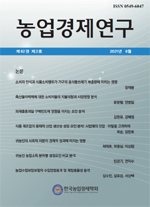상세검색
최근 검색어 전체 삭제
다국어입력
즐겨찾기0KCI등재 학술저널

# 귀농인 농업소득 분위별 결정요인 비교 분석A Comparative Analysis on the Determinants of Agricultural Income of Returning Farmers by Quantile

• 등재여부 : KCI등재
• 2021.06
• 111 - 134 (24 pages)
• 11

The purpose of this paper is to compare and analyze the determinants of agricultural income by quantile through quantile regression considering the heterogeneous distribution of agricultural income of returning farmers. Unlike OLS of the mean concept, quantile regression is an analysis method that can consider the entire distribution, including each quantile. In this study, the 25th, 50th, and 75th quartiles were analyzed and compared to each other and they were compared with the OLS result. The results showed that 15 factors in the OLS result, 4 factors in the 25th quartile, 9 factors in the 50th quartile, and 14 factors in the 75th quartile were found to have a statistically significant effect. This can leads to a conclusion that the OLS result, which is based on the average concept, was relatively more influenced by high quantiles such as the 75th quartile. In other words, this implies that the characteristics of low-income return farmers were relatively less reflected. The magnitude of the influence also showed a similar tendency to increase with higher quantiles.

Ⅰ. 서론

Ⅱ. 이론적 배경 및 분석방법

Ⅲ. 분석 결과

Ⅳ. 요약 및 결론